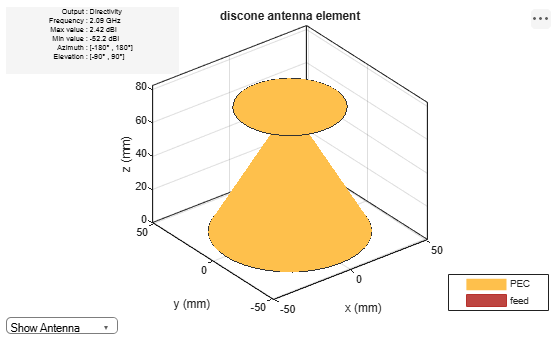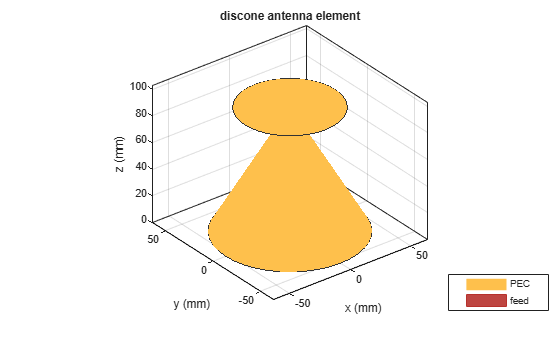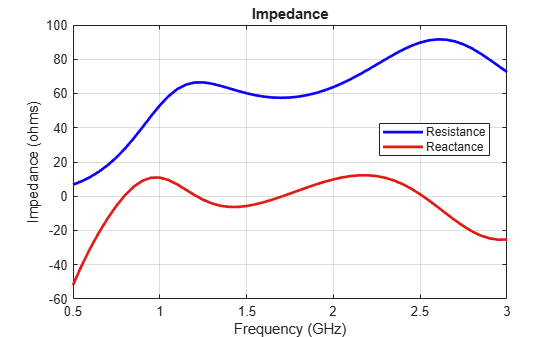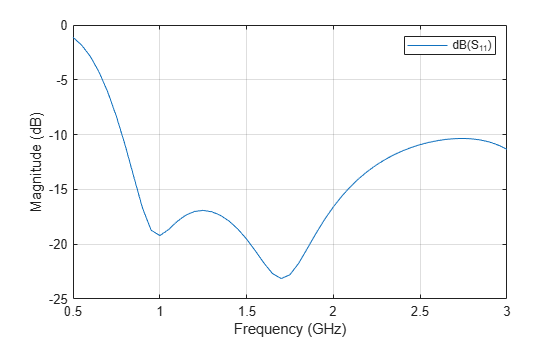# discone

Create discone antenna

## Description

The `discone` object creates a discone antenna that consists of a circular disc and a cone whose apex approaches the center of the disc. A small gap exists between the disc and the cone through which the feed is connected.

A discone antenna is an omnidirectional vertically polarized antenna. This antenna has an exceptionally large coverage, offering a frequency range ratio of up to 10:1 between the upper cutoff frequency and the lower cutoff frequency. The discone antenna wideband coverage makes it useful in commercial, military, amateur radio, and radio scanner applications.## Creation

### Syntax

``ant = discone``
``ant = discone(Name,Value)``

### Description

example

````ant = discone` creates a discone antenna with dimensions for a resonant frequency of 2.09 GHz. The default discone has a feedpoint at the center of the disc.```

example

````ant = discone(Name,Value)` sets properties using one or more name-value pairs. For example, ```ant = discone('Height',1)``` creates a discone antenna with a cone of height 1 meter.```

### Output Arguments

expand all

Discone antenna, returned as a `discone` object.

## Properties

expand all

Vertical height of the cone from the center of the lower base of the cone to the center of the upper base of the cone, specified as a real-valued scalar in meters.

Example: `'Height',1`

Example: `ant.Height = 1`

Data Types: `double`

Radii of the cone consisting of the broad radius and the narrow radius, specified as a vector with each element unit in meters. The first element of the vector is the narrow radius, and the second element of the vector is the broad radius.

Example: `'ConeRadii',[6.3300e-04 0.0546]`

Example: ```ant.ConeRadii = [6.3300e-04 0.0546]```

Data Types: `double`

Radius of the disc, specified as a real-valued scalar in meters.

Example: `'DiscRadius',0.0050`

Example: `ant.DiscRadius = 0.050`

Data Types: `double`

Gap between the cone and the disc, specified as a real-valued scalar in meters.

Example: `'FeedHeight',0.0034`

Example: `ant.FeedHeight = 0.0034`

Data Types: `double`

Diameter of the feed, specified as a real-valued scalar in meters.

Example: `'FeedWidth',0.0050`

Example: `ant.FeedWidth = 0.0050`

Data Types: `double`

Lumped elements added to the antenna feed, specified as a lumped element object handle. You can add a load anywhere on the surface of the antenna. By default, the load is at the feed. For more information, see `lumpedElement`.

Example: `'Load',lumpedelement`. `lumpedelement` is the object handle for the load created using `lumpedElement`.

Example: ```ant.Load = lumpedElement('Impedance',75)```

Tilt angle of the antenna, specified as a scalar or vector with each element unit in degrees. For more information, see Rotate Antennas and Arrays.

Example: `'Tilt',90`

Example: `'Tilt',[90 90]`,`'TiltAxis',[0 1 0;0 1 1]` tilts the antenna at 90 degree about two axes, defined by vectors.

Data Types: `double`

Tilt axis of the antenna, specified as:

• Three-element vectors of Cartesian coordinates in meters. In this case, each vector starts at the origin and lies along the specified points on the X-, Y-, and Z-axes.

• Two points in space, each specified as three-element vectors of Cartesian coordinates. In this case, the antenna rotates around the line joining the two points in space.

• A string input describing simple rotations around one of the principal axes, 'X', 'Y', or 'Z'.

Example: `'TiltAxis',[0 1 0]`

Example: `'TiltAxis',[0 0 0;0 1 0]`

Example: `ant.TiltAxis = 'Z'`

## Object Functions

 `show` Display antenna or array structure; Display shape as filled patch `axialRatio` Axial ratio of antenna `beamwidth` Beamwidth of antenna `charge` Charge distribution on metal or dielectric antenna or array surface `current` Current distribution on metal or dielectric antenna or array surface `design` Design prototype antenna or arrays for resonance at specified frequency `EHfields` Electric and magnetic fields of antennas; Embedded electric and magnetic fields of antenna element in arrays `impedance` Input impedance of antenna; scan impedance of array `mesh` Mesh properties of metal or dielectric antenna or array structure `meshconfig` Change mesh mode of antenna structure `pattern` Radiation pattern and phase of antenna or array; Embedded pattern of antenna element in array `patternAzimuth` Azimuth pattern of antenna or array `patternElevation` Elevation pattern of antenna or array `returnLoss` Return loss of antenna; scan return loss of array `sparameters` S-parameter object `vswr` Voltage standing wave ratio of antenna

## Examples

collapse all

Create and view a default discone antenna.

```ant = discone; show(ant)```Plot the radiation pattern of the antenna at 2.09 GHz.

`pattern(ant,2.09e9)`Create and view a discone antenna with specific dimensions.

```ant = discone('Height',0.0925,'ConeRadii',[0.666e-3 53.2e-3],... 'DiscRadius',37.25e-3,'FeedHeight',399.7e-6,'FeedWidth',0.553e-3); show(ant) ```Calculate the impedance of the antenna over the frequency span of 500 MHz to 3 GHz and plot the S-parameters.

`impedance(ant,linspace(0.5e9,3e9,51));````s = sparameters(ant,linspace(0.5e9,3e9,51)); figure; rfplot(s);```Plot the radiation pattern of the antenna at 1.7 GHz.

`pattern(ant,1.7e9);`Verma, Saritha, Abhilash Mehta, and Rukhsana Khan. "Analysis of Variation of Various Parameters on Design of Discone Antenna." Advanced Computational Techniques in Electromagnetics. Volume 2012, 2012, pp.1-5.